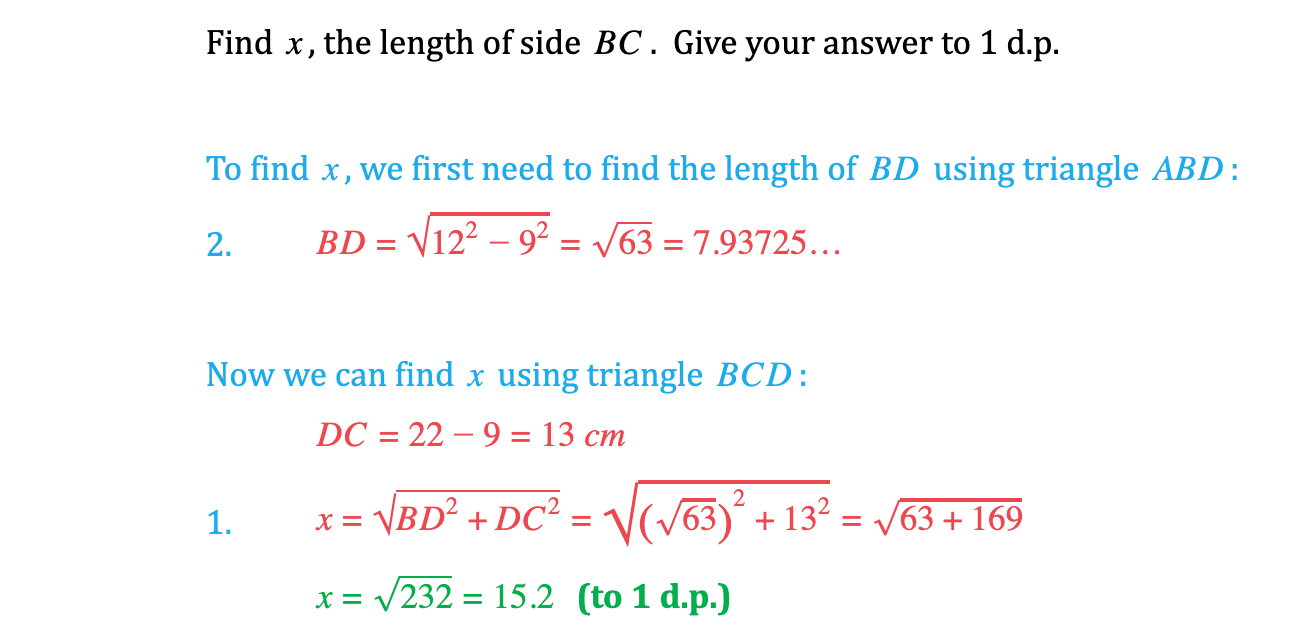# Edexcel IGCSE Maths 复习笔记 4.8.1 Pythagoras Theorem

Edexcel IGCSE Maths 复习笔记 4.8.1 Pythagoras Theorem

#### What is Pythagoras?

• Pythagoras was a Greek mathematician who lived over 2500 years ago
• He is most famous for Pythagoras’ Theorem, which includes the important formula for right-angled triangles

#### Pythagoras' theorem?

• The longest side in a right-angled triangle is called the hypotenuse – this will always be the side opposite the right angle
• If we label the hypotenuse c, and label the other two sides a and b:then Pythagoras’ Theorem tells us that:

a2 + b2 = c2

(where a, b, and c are the lengths of the three sides)

#### Using Pythagoras’ theorem

• To find the length of the hypotenuse use:c = √a2 + b2

(Note that when finding the hypotenuse you add inside the square root)

• To find the length of one of the other sides use:

a = √c2 – b2 or b = √c2 – a2Note that when finding one of the other sides you subtract inside the square root

#### Exam Tip

‘Reality check’ your answers.  If the hypotenuse ends up being shorter than another side in your answer, you’ve made a mistake somewhere!If your calculator gives you a ‘Math ERROR’ result, you probably subtracted the wrong way around in the square root.In questions with multiple steps, don’t round off (to 1 decimal place, 3 significant figures, etc) until the very end.

#### Worked Example#### Worked Example